# ISEE Middle Level Quantitative : Geometry

## Example Questions

### Example Question #2 : Plane Geometry

If a square has sides measuring, what is the perimeter of the square, in simplest form?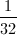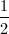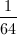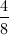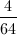Explanation:

To find the perimeter of a square, you must add together all the sides. In this case, we are addingfour times.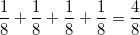Since all of the denominators are the same, there is no need to find a commond denominator, so we add together the numerators.  This gives us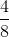.

Since both the numerator and denomator are divisible by four, we must simplify this fraction.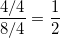The perimeter of the square is.

### Example Question #21 : Geometry

The sum of the lengths of three sides of a square is one foot. Give the perimeter of the square in inches.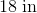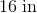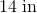It is impossible to determine the perimeter from the information given.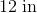Explanation:

A square has four sides of the same length.

One foot is equal to twelve inches; since the sum of the lengths of three of the congruent sides is twelve inches, each side measures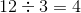inches.

The perimeter is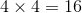inches.

### Example Question #22 : Geometry

A square has perimeter one yard. Which is the greater quantity?

(A) The length of one side of the square

(B) 8 inches

(B) is greater

(A) is greater

It is impossible to determine which is greater from the information given

(A) and (B) are equal

(A) is greater

Explanation:

One yard is equal to 36 inches. A square has four sides of equal length, so one side of the square has length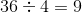inches.

Since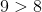, (A) is greater.

### Example Question #23 : Geometry

A square has perimeter five meters. Which is the greater quantity?

(A) 1,250 millimeters

(B) The length of one side of the square

(B) is greater

(A) is greater

(A) and (B) are equal

It is impossible to determine which is greater from the information given

(A) and (B) are equal

Explanation:

One meter is equal to 1,000 millimeters, so the square has perimeter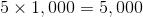millimeters.

A square has four sides of equal length, so one side of the square has length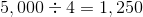millimeters.

The quantities are equal.

### Example Question #24 : Geometry

A square lot has perimeter one mile. Which is the greater quantity?

(A) 1,320 feet

(B) The length of one side of the square

(A) and (B) are equal

(B) is greater

It is impossible to tell which is greater from the information given

(A) is greater

(A) and (B) are equal

Explanation:

One mile is equal to 5,280 feet. A square has four sides of equal length, so one side of the square has length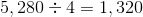feet.

The quantities are equal.

### Example Question #25 : Geometry

The sum of the lengths of three sides of a square is 9,000 centimeters. Give its perimeter in meters.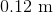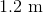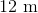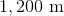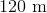Explanation:

100 centimeters are equal to one meter, so 9,000 centimeters are equal to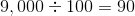meters.

A square has four sides of the same length. Since the sum of the lengths of three of the congruent sides is 9,000 centimeters, or 90 meters, each side measures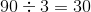meters.

The perimeter is four times this, which is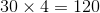meters.

### Example Question #26 : Geometry

A square has perimeter one meter. Which is the greater quantity?

(A) 250 centimeters

(B) The length of one side of the square

(A) is greater

(B) is greater

It is impossible to tell which is greater from the information given

(A) and (B) are equal

(A) is greater

Explanation:

One meter is equal to 100 centimeters.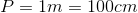A square has four sides of equal length, so we will need to divide by 4 to find the length of one side.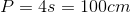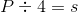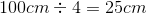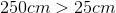, so (A) is greater

### Example Question #27 : Geometry

Each side of a square is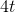units long. Which is the greater quantity?

(A)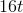(B) The perimeter of the square

(A) and (B) are equal

(A) is greater

(B) is greater

It is impossible to determine which is greater from the information given

(A) and (B) are equal

Explanation:

The perimeter of a square is four times its side length: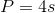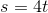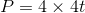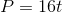Since the perimeter is equal to, (A) and (B) are equal.

### Example Question #28 : Geometry

Hexagon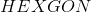and Square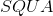both have perimeter 64. Which is the greater quantity?

(a)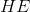(b)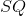It is impossible to determine which is greater from the information given

(b) is the greater quantity

(a) and (b) are equal

(a) is the greater quantity

It is impossible to determine which is greater from the information given

Explanation:

The length of one side of the square - in particular, that of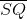- is equal to one fourth its perimeter, so it can be determined that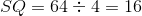. However, no relation among the sides of the hexagon is given - in particular, it is not given that the hexagon is regular - so the length of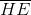cannot be determined. Insufficient information is given.

### Example Question #29 : Geometry

What is the length of a rectangular room with a perimeter of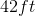and a width of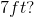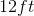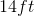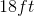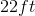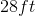Explanation: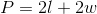We have the perimeter and the width, so we can plug those values into our equation and solve for our unknown.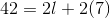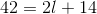Subtractfrom both sides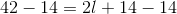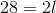Divideby both sides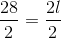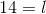### All ISEE Middle Level Quantitative Resources